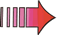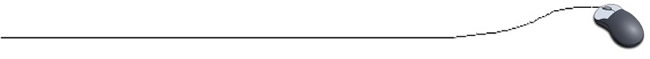Initializing and Printing Arrays

When an array is declared, a sufficient amount of memory is set aside to hold the elements. The array is then initialized to the default values for the element type.
For example,
• if the elements in the array are integers (int), each element in the array will be automatically initialized to 0.
• if the elements in the array are Strings, each element will be initialized to the null character (null).
• if the elements in the array are boolean, each element will be initialized to false.
Now, let's see the options for initializing an array with data that we will be using in our program.

Ways to initialize an array:Using the assignment operator (=) to initialize an array (the "drudge" method):

int [ ] temps = new int [ 3 ];
temps = 78;  //filling one element at a time
temps = 88;
temps = 53;

Works fine until the array needs to contain a large amount of data.Using a for loop and user input to initialize an array:

int [ ] nums = new int [ 8 ];
for(int ctr = 0; ctr < 8; ctr++)
{
//fill one element at a time
System.out.println("Enter number: ");
}Initialize at time of declaration - filling by list. It is possible to fill an array at the time of declaration.

double [ ]  temperature = {13.5, 18.4, 19.6, 21.4};

The array length (size) will be automatically set to the minimum that will hold the given values.  The statement above is equivalent to the following statements:

double [ ] temperature = new double ;
temperature = 13.5;
temperature = 18.4;
temperature = 19.6;
temperature = 21.4;Declare and initialize an array of strings:

//fill by list
String [ ] dogs = {"Henry", "Lucy", "Josie"};

// fill one element at a time with user input
String [ ] list = new String ;
for ( i = 0; i < list.length; i++)
{

System.out.println("Enter number: ");
list[ i ] = reply.nextLine( );
}

Printing an array:Use a for loop for printing arrays:

for(int i = 0; i < dogs.length; i++)
{
//print one element at a time
System.out.println(dogs[ i ]);
}Use an enhanced for loop (called the for-each loop) especially designed to print each element in an array:

for (type variableName : arrayName) // this syntax does not need a loop control variable
{
. . .
}

//Example
for(String element : dogs)
{
//print one element at a time
System.out.println(element);
}
Note: the for-each loop was introduced in Java 5 (JDK 5). While this process is new and novel, it can only be used to print the array from index 0 to the end of the array. You cannot control the index of the array. For example, you cannot print the array in reverse order using this syntax.

Changing an array element:Use the assignment operator (=) to change an element in an array:

If you wish to change an element in an established array, simply use "assignment".
For example, if you want to change the second element in the temperature array (seen above) to 30.4, use

temperature = 30.4;